### Example Workflow For Processing a Single Screen

This is an example workflow for processing a pooled screening eperiment using the provided sample data. See the various manpages for additional visualization options and algorithmic details.

Note that what follows describes a very basic analysis. If you are considering integrating the results of many different screen contrasts or even different experiments and/or technologies, refer to the `Advanced Screen Analysis: Contrast Comparisons` vignette.

``````suppressPackageStartupMessages(library(Biobase))
suppressPackageStartupMessages(library(limma))
suppressPackageStartupMessages(library(gCrisprTools))

data("es", package = "gCrisprTools")
data("ann", package = "gCrisprTools")
data("aln", package = "gCrisprTools")
knitr::opts_chunk\$set(message = FALSE, fig.width = 8, fig.height = 8, warning = FALSE)
``````

Make a sample key, structured as a factor with control samples in the first level

``````sk <- relevel(as.factor(pData(es)\$TREATMENT_NAME), "ControlReference")
names(sk) <- row.names(pData(es))
``````

Generate a contrast of interest using voom/limma; pairing replicates is a good idea if that information is available.

``````design <- model.matrix(~ 0 + REPLICATE_POOL + TREATMENT_NAME, pData(es))
colnames(design) <- gsub('TREATMENT_NAME', '', colnames(design))
contrasts <-makeContrasts(DeathExpansion - ControlExpansion, levels = design)
``````

Optionally, trim of trace reads from the unnormalized object (see man page for details)

``````es <- ct.filterReads(es, trim = 1000, sampleKey = sk)
``````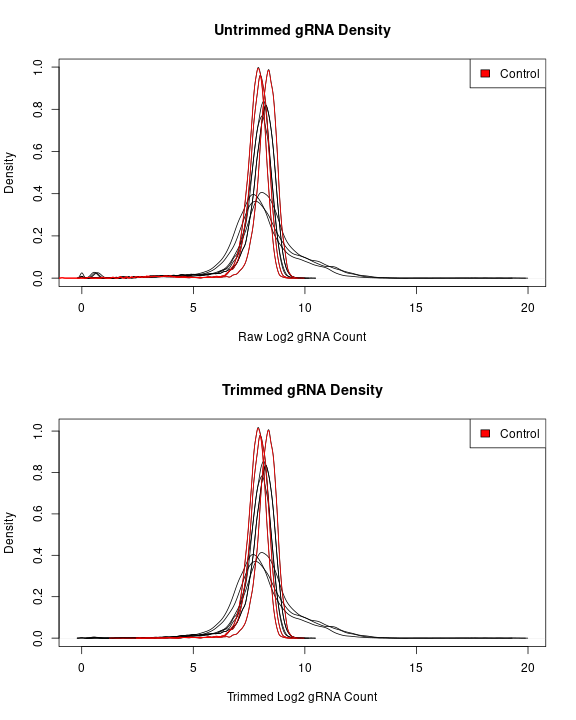Normalize, convert to a voom object, and generate a contrast

``````es <- ct.normalizeGuides(es, method = "scale", plot.it = TRUE) #See man page for other options
vm <- voom(exprs(es), design)

fit <- lmFit(vm, design)
fit <- contrasts.fit(fit, contrasts)
fit <- eBayes(fit)
``````

Edit the annotation file if you used `ct.filterReads` above

``````ann <- ct.prepareAnnotation(ann, fit, controls = "NoTarget")
``````

Summarize gRNA signals to identify target genes of interest

``````resultsDF <-
ct.generateResults(
fit,
annotation = ann,
RRAalphaCutoff = 0.1,
permutations = 1000,
scoring = "combined",
permutation.seed = 2
)
``````

#### Alternative Annotations

In some cases, reagents might target multiple known elements (e.g., gRNAs in a CRISPRi library that target multiple promoters of the same gene). In such cases, you can specify this via the `alt.annotation` argument to `ct.generateResults()`. Alternative annotations are supplied as a list of character vectors named for the reagents.

``````# Create random alternative target associations

altann <- sapply(ann\$ID,
function(x){
out <- as.character(ann\$geneSymbol)[ann\$ID %in% x]
if(runif(1) < 0.01){out <- c(out, sample(as.character(ann\$geneSymbol), size = 1))}
return(out)
}, simplify = FALSE)

resultsDF <-
ct.generateResults(
fit,
annotation = ann,
RRAalphaCutoff = 0.1,
permutations = 1000,
scoring = "combined",
alt.annotation = altann,
permutation.seed = 2
)
``````

Optionally, just load an example results object for testing purposes (trimming out reads as necessary)

``````data("fit", package = "gCrisprTools")
data("resultsDF", package = "gCrisprTools")

fit <- fit[(row.names(fit) %in% row.names(ann)),]
resultsDF <- resultsDF[(row.names(resultsDF) %in% row.names(ann)),]

targetResultDF <- ct.simpleResult(resultsDF) #For a simpler target-level result object
``````

### Quality Control

gCrisprTools contains a variety of pooled screen-specific quality control and visualization tools (see man pages for details):

``````ct.alignmentChart(aln, sk)
``````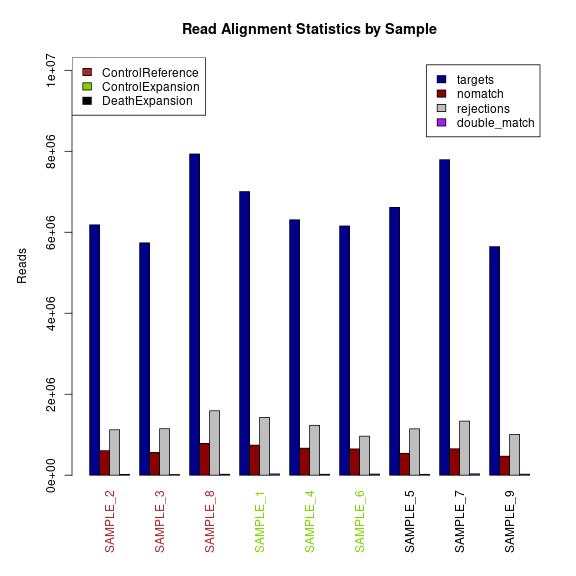``````ct.rawCountDensities(es, sk)
``````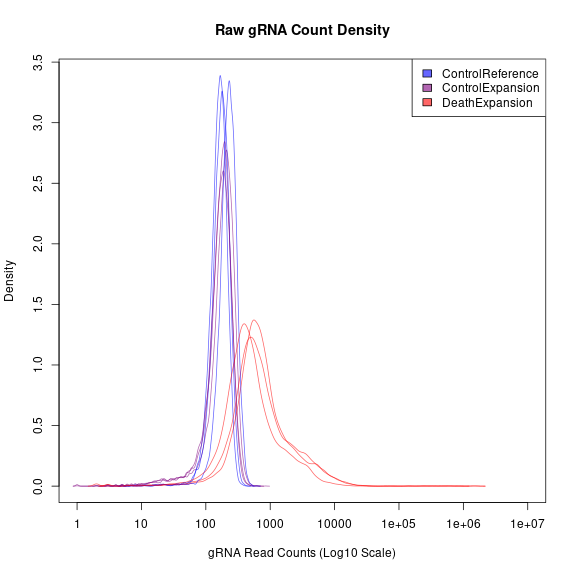Visualize gRNA abundance distributions

``````ct.gRNARankByReplicate(es, sk)
``````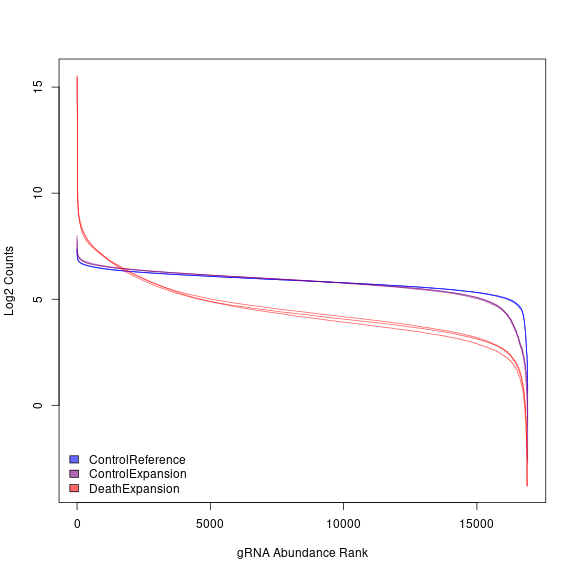``````ct.gRNARankByReplicate(es, sk, annotation = ann, geneSymb = "NoTarget")  #Show locations of NTC gRNAs
``````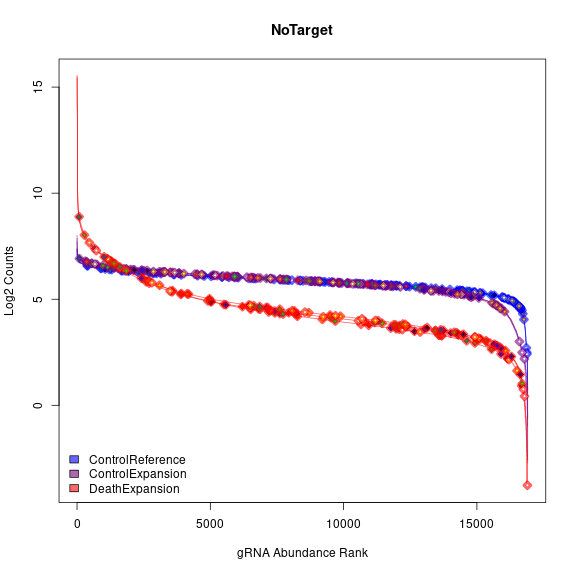Visualize control guide behavior across conditions

``````ct.viewControls(es, ann, sk, normalize = FALSE)
``````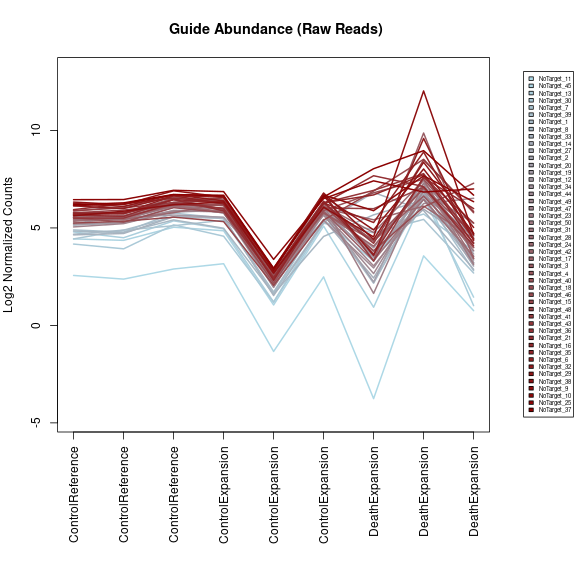``````ct.viewControls(es, ann, sk, normalize = TRUE)
``````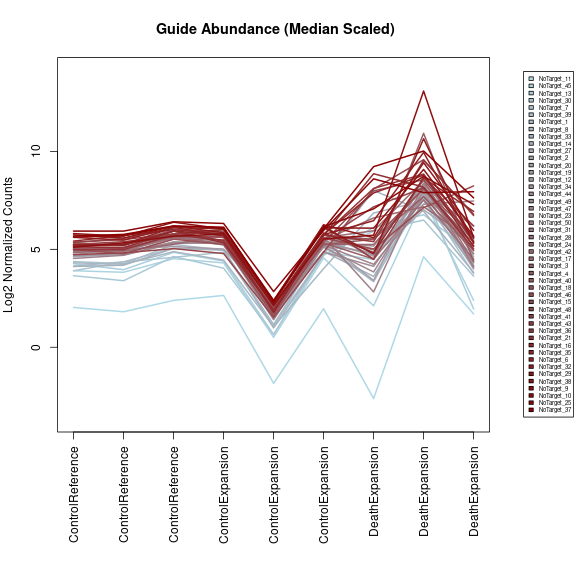Visualize GC bias across samples, or within an experimental contrast

``````ct.GCbias(es, ann, sk)
``````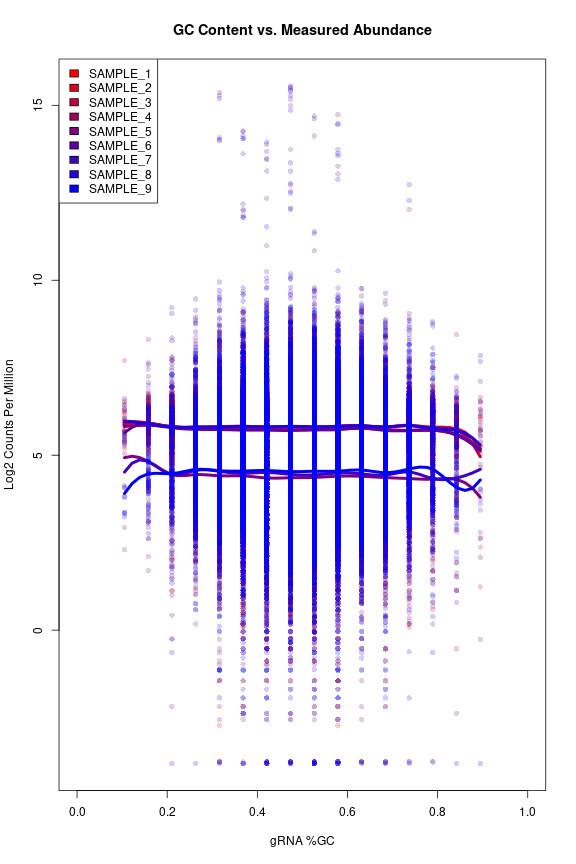``````ct.GCbias(fit, ann, sk)
``````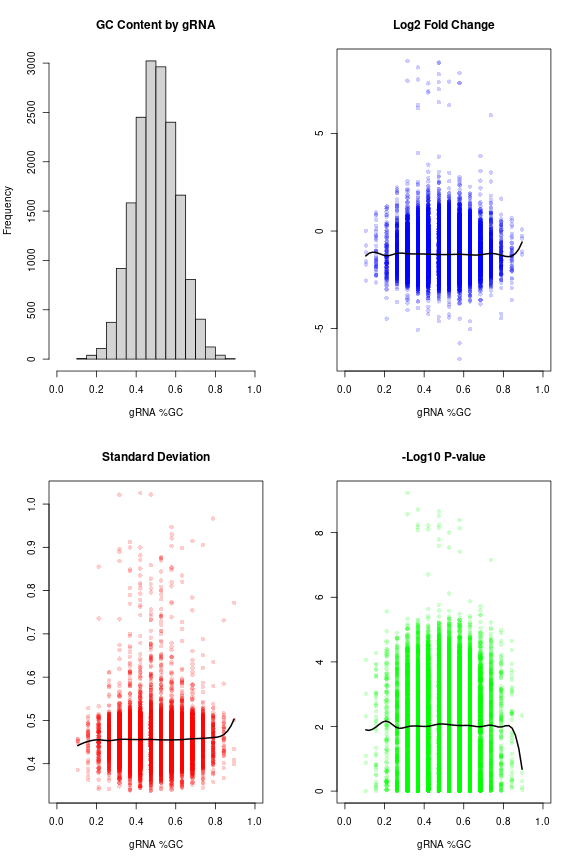View most variable gRNAs/Genes (as % of sequencing library)

``````ct.stackGuides(es,
sk,
plotType = "gRNA",
annotation = ann,
nguides = 40)
``````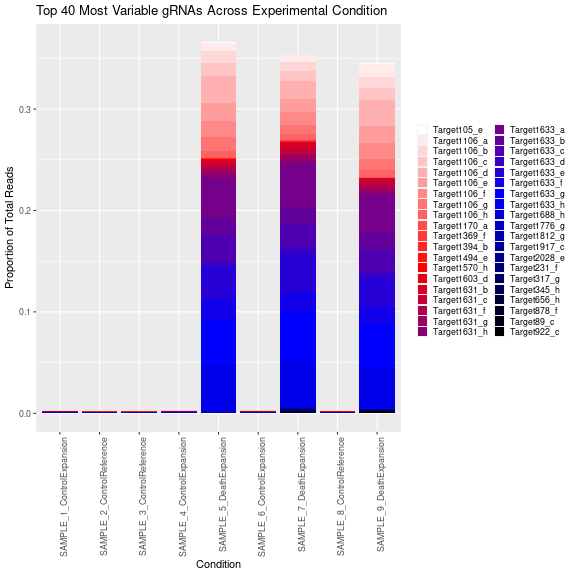``````ct.stackGuides(es,
sk,
plotType = "Target",
annotation = ann)
``````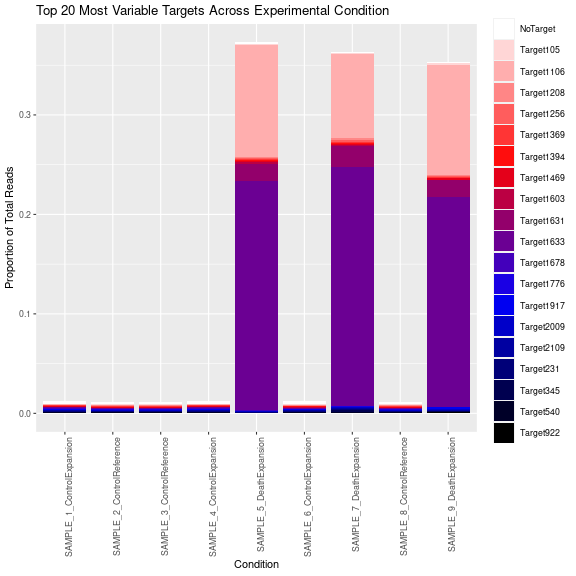``````ct.stackGuides(es,
sk,
plotType = "Target",
annotation = ann,
subset = names(sk)[grep('Expansion', sk)])
``````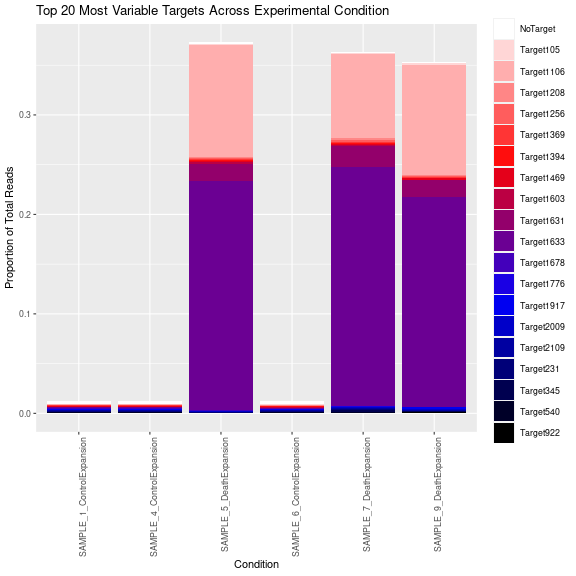View a CDF of genes/guides

``````ct.guideCDF(es, sk, plotType = "gRNA")
``````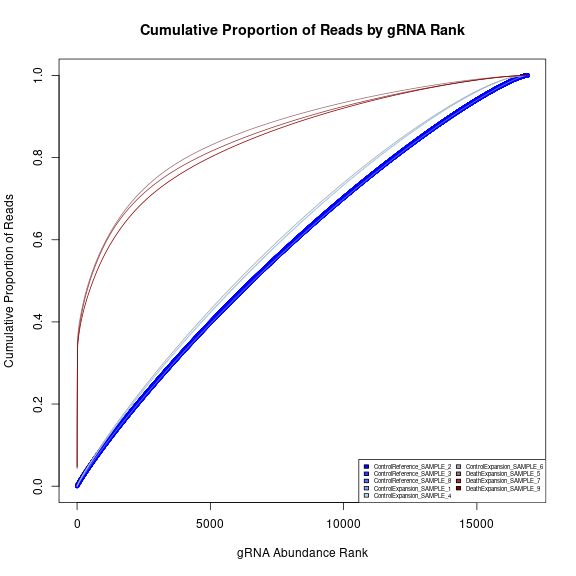``````ct.guideCDF(es, sk, plotType = "Target", annotation = ann)
``````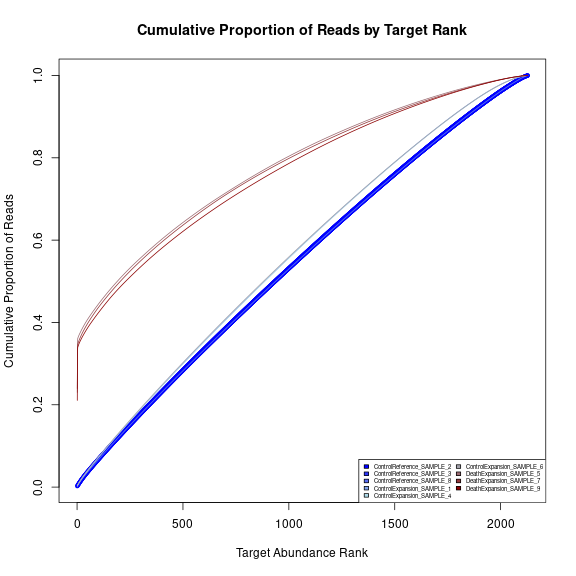#### Target-Level Visualization and Analysis

View the overall enrichment and depletion trends identified in the screen:

``````ct.contrastBarchart(resultsDF)
``````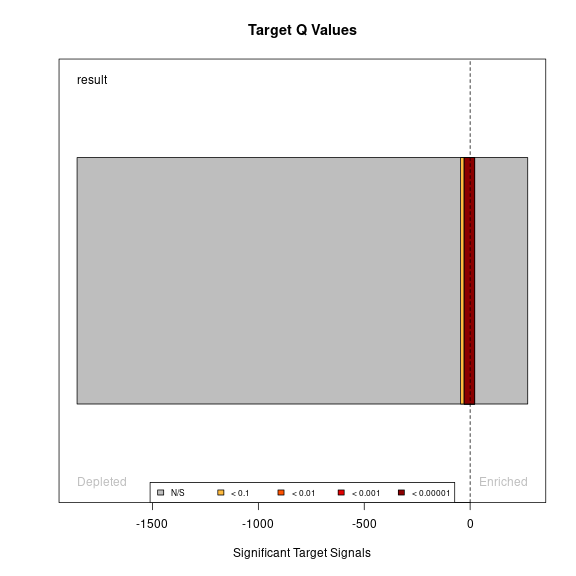View top enriched/depleted candidates

``````ct.topTargets(fit,
resultsDF,
ann,
targets = 10,
enrich = TRUE)
``````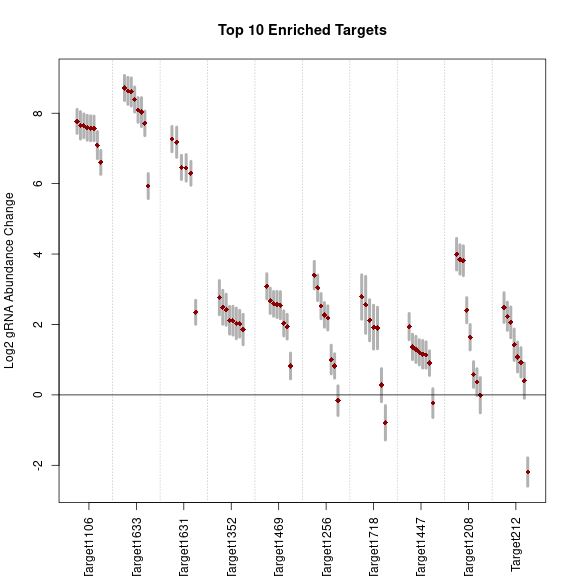``````ct.topTargets(fit,
resultsDF,
ann,
targets = 10,
enrich = FALSE)
``````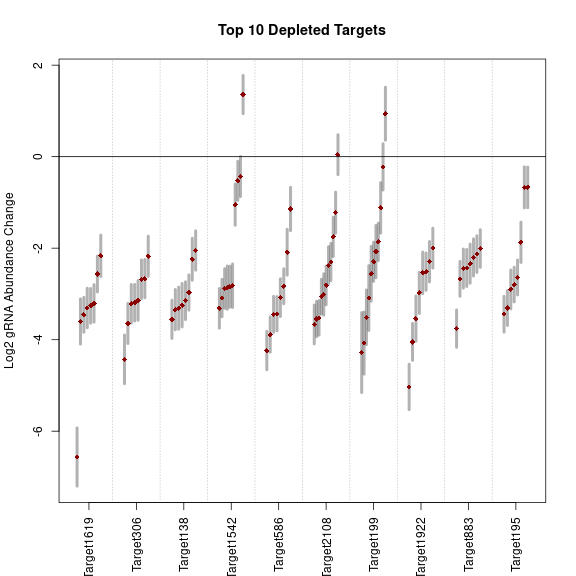View the behavior of reagents targeting a particular gene of interest

``````ct.viewGuides("Target1633", fit, ann)
``````